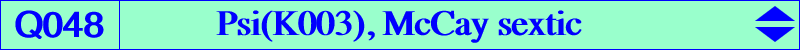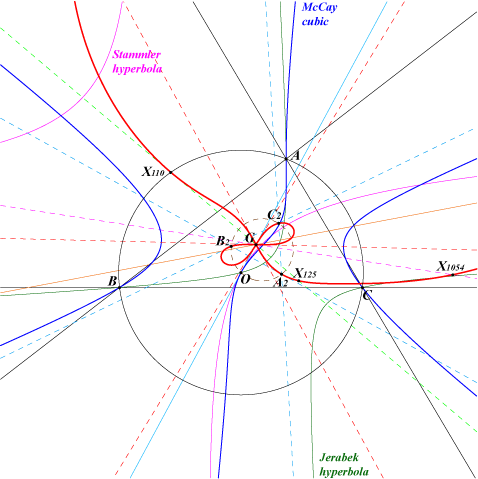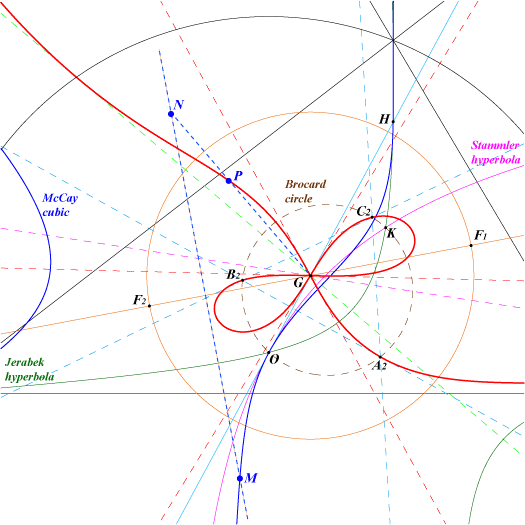too complicated to be written here. Click on the link to download a text file.X(2), X(110), X(125), X(1054) A2, B2, C2 : vertices of the second Brocard triangle extraversions of X(1054)Since the McCay cubic is an equilateral cubic, the polar conic of any point M of the plane is a rectangular hyperbola with center P. The mapping M -> P is denoted Psi in "Orthocorrespondence and Orthopivotal Cubics", §5, page 10. See the Downloads page. If N is the reflection of M in the focal axis of the Steiner inellipse then P is the inverse of N in the circle with diameter F1F2 (F1, F2 are the foci of the Steiner inellipse). Psi is clearly an involution. The locus of P when M traverses the McCay cubic is Q048, the McCay sextic. See the analogous Q041, Q119. Q048 is a tricircular sextic passing through G which is a triple point on the curve. The three tangents at G make 60° angles with one another. Q048 also contains : - X(110), the center of the Stammler hyperbola which is the polar conic of O, - X(125), the center of the Jerabek hyperbola which is the polar conic of H, - X(1054), the center of the hyperbola which is the polar conic of I, and the extraversions of X(1054) obtained with the excenters, - A2, B2, C2, the vertices of the second Brocard triangle, centers of the polar conics of A, B, C. Since Psi transforms the circumcircle into the Brocard circle, the vertices of the circumnormal triangle give the last three other common points of Q048 and the Brocard circle. The Psi-transform of the sideline BC is the circle passing through G, B2, C2. This circle meets Q048 again at the Psi-image of the trace of O on BC.